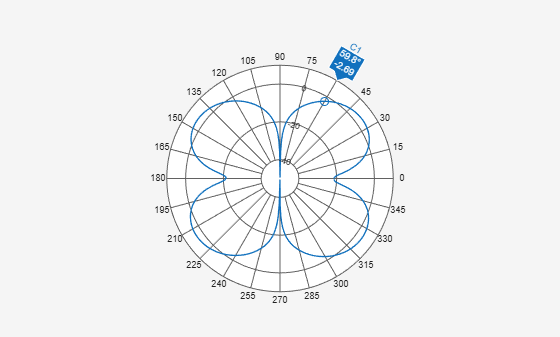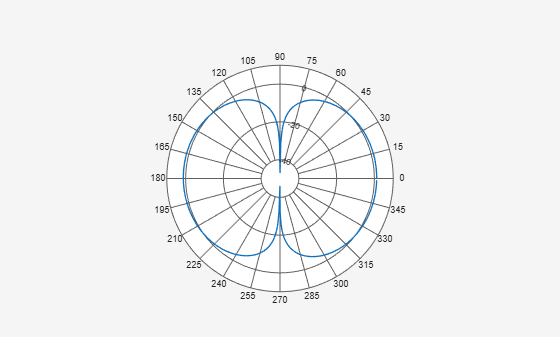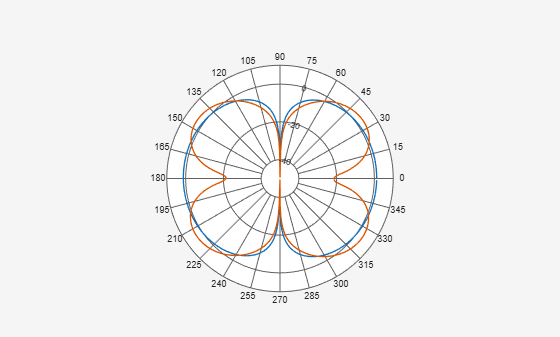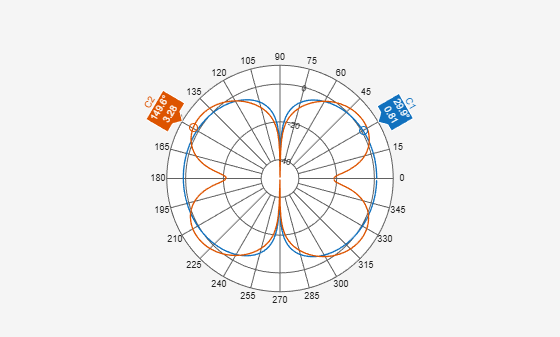Class: polarpattern

Add cursor to polar plot angle

## Syntax

``addCursor(p,angle)``
``addCursor(p,angle,index)``
``id = addCursor(___)``

## Description

example

````addCursor(p,angle)` adds a cursor to the active polar plot, `p`, at the data point closest to the specified `angle`. Angle units are in degrees.The first cursor added is called `'C1'`, the second `'C2'`, and so on.```

example

````addCursor(p,angle,index)` adds a cursor at a specified data set `index`. `index` can be a vector of indices.```
````id = addCursor(___)` returns a cell array with one ID for each cursor created. You can specify any of the arguments from the previous syntaxes.```

## Input Arguments

expand all

Polar plot, specified as a scalar handle.

Angle values at which the cursor is added, specified as a scalar or a vector in degrees.

Data set index, specified as a scalar or a vector.

## Examples

expand all

Create a dipole antenna and calculate its directivity at a frequency of 270 MHz.

```d = dipole; D = pattern(d,270e6,0,0:1:360);```

Add a cursor to the polar plot at approximately 60 degrees. To place the cursor at 60 degrees, move it there by placing the pointer on the cursor and dragging.

```p = polarpattern(D); addCursor(p,60);```Create a top-hat monopole and plot its directivity at a frequency of 75 MHz.

```m = monopoleTopHat; M = pattern(m,75e6,0,0:1:360); P = polarpattern(M);```Create a dipole antenna and calculate its directivity at a frequency of 270 MHz.

```d = dipole; D = pattern(d,270e6,0,0:1:360);```

Add the directivity pattern of the dipole to the polar plot of the top-hat monopole.

`add(P,D);`Add a cursor at approximately 30 degrees to the top-hat monopole polar pattern (data set 1) and at approximately 150 degrees to the dipole polar pattern (data set 2).

`addCursor(P,[30 150],[1 2]);`## Version History

Introduced in R2016a